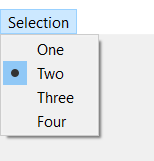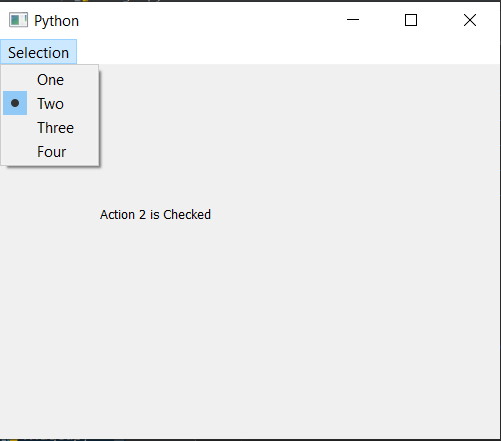# PyQt5 – QActionGroup

• Last Updated : 01 Feb, 2022

QActionGroup : In PyQt5 applications many common commands can be invoked via menus, toolbar buttons, and keyboard shortcuts, since the user expects each command to be performed in the same way, regardless of the user interface used, QAction is useful to represent each command as an action. In some situations it is useful to group QAction objects together, so that user can only select(check) only one QAction at a time just like radio buttons. Also in order to videw the effect og action group the action added to it should be checkable.
Below is how an actions in action group will look inside the menuSyntax:

`action_group = QActionGroup()`

This action_group is used by adding those QAction which should lie in same group, they can be added with the help of addAction method. Below are the some frequently used commands with the QAction

```addAction : To add QAction to it

setEnabled : To make QActionGroup enable or disable

setExclusionPolicy : To set exclusion policy to the action group

checkedAction : It returns the currently checked action

removeAction : To remove the specific QAction from the group

actions : It returns the list of QAction group is having```

Example :
In this we will create a menu bar which will have a menu, and having multiple checkable QAction, below is the implementation

## Python3

 `# importing libraries``from` `PyQt5.QtWidgets ``import` `*` `from` `PyQt5 ``import` `QtCore, QtGui``from` `PyQt5.QtGui ``import` `*` `from` `PyQt5.QtCore ``import` `*` `import` `sys`  `class` `Window(QMainWindow):` `    ``def` `__init__(``self``):``        ``super``().__init__()` `        ``# setting title``        ``self``.setWindowTitle("Python ")` `        ``# setting geometry``        ``self``.setGeometry(``100``, ``100``, ``500``, ``400``)` `        ``# calling method``        ``self``.UiComponents()` `        ``# showing all the widgets``        ``self``.show()`   `    ``# method for components``    ``def` `UiComponents(``self``):` `        ``# creating a menu bar``        ``menubar ``=` `self``.menuBar()` `        ``# creating a selection menu``        ``selMenu ``=` `menubar.addMenu(``'Selection'``)` `        ``# creating QAction Instances``        ``action1 ``=` `QAction("One", ``self``)``        ``action2 ``=` `QAction("Two", ``self``)``        ``action3 ``=` `QAction("Three", ``self``)``        ``action4 ``=` `QAction("Four", ``self``)` `        ``# making actions checkable``        ``action1.setCheckable(``True``)``        ``action2.setCheckable(``True``)``        ``action3.setCheckable(``True``)``        ``action4.setCheckable(``True``)` `        ``# adding these actions to the selection menu``        ``selMenu.addAction(action1)``        ``selMenu.addAction(action2)``        ``selMenu.addAction(action3)``        ``selMenu.addAction(action4)` `        ``# creating a action group``        ``action_group ``=` `QActionGroup(``self``)` `        ``# adding these action to the action group``        ``action_group.addAction(action1)``        ``action_group.addAction(action2)``        ``action_group.addAction(action3)``        ``action_group.addAction(action4)` `        ``# creating a label``        ``label ``=` `QLabel("GeeksforGeeks", ``self``)` `        ``# setting geometry to the label``        ``label.setGeometry(``100``, ``150``, ``200``, ``50``)` `        ``# adding triggered action to the first action``        ``action1.triggered.connect(``lambda``: label.setText("Action ``1` `is` `Checked"))` `        ``# adding triggered action to the second action``        ``action2.triggered.connect(``lambda``: label.setText("Action ``2` `is` `Checked"))` `        ``# adding triggered action to the third action``        ``action3.triggered.connect(``lambda``: label.setText("Action ``3` `is` `Checked"))` `        ``# adding triggered action to the fourth action``        ``action4.triggered.connect(``lambda``: label.setText("Action ``4` `is` `Checked"))`    `# create pyqt5 app``App ``=` `QApplication(sys.argv)` `# create the instance of our Window``window ``=` `Window()` `# start the app``sys.exit(App.``exec``())`

Output :Another Example
In this we will create a tool bar having multiple QAction which are dived by two action group, below is the implementation

## Python3

 `# importing libraries``from` `PyQt5.QtWidgets ``import` `*` `from` `PyQt5 ``import` `QtCore, QtGui``from` `PyQt5.QtGui ``import` `*` `from` `PyQt5.QtCore ``import` `*` `import` `sys`  `class` `Window(QMainWindow):` `    ``def` `__init__(``self``):``        ``super``().__init__()` `        ``# setting title``        ``self``.setWindowTitle("Python ")` `        ``# setting geometry``        ``self``.setGeometry(``100``, ``100``, ``500``, ``400``)` `        ``# calling method``        ``self``.UiComponents()` `        ``# showing all the widgets``        ``self``.show()` `        ``# method for components` `    ``def` `UiComponents(``self``):``        ``# creating a tool bar``        ``toolbar ``=` `QToolBar(``self``)` `        ``# setting geometry to the tool bar``        ``toolbar.setGeometry(``50``, ``100``, ``300``, ``35``)` `        ``# creating QAction Instances``        ``action1 ``=` `QAction("One", ``self``)``        ``action2 ``=` `QAction("Two", ``self``)``        ``action3 ``=` `QAction("Three", ``self``)``        ``action4 ``=` `QAction("Four", ``self``)` `        ``# making actions checkable``        ``action1.setCheckable(``True``)``        ``action2.setCheckable(``True``)``        ``action3.setCheckable(``True``)``        ``action4.setCheckable(``True``)` `        ``# adding these actions to the tool bar``        ``toolbar.addAction(action1)``        ``toolbar.addAction(action2)``        ``toolbar.addAction(action3)``        ``toolbar.addAction(action4)` `        ``# creating a first action group``        ``action_group1 ``=` `QActionGroup(``self``)` `        ``# adding these action to the action group``        ``action_group1.addAction(action1)``        ``action_group1.addAction(action2)` `        ``# creating a second action group``        ``action_group2 ``=` `QActionGroup(``self``)` `        ``action_group2.addAction(action3)``        ``action_group2.addAction(action4)` `        ``# creating a label``        ``label ``=` `QLabel("GeeksforGeeks", ``self``)` `        ``# setting geometry to the label``        ``label.setGeometry(``100``, ``150``, ``200``, ``50``)` `        ``# adding triggered action to the first action``        ``action1.triggered.connect(``lambda``: label.setText("Action ``1` `is` `Checked"))` `        ``# adding triggered action to the second action``        ``action2.triggered.connect(``lambda``: label.setText("Action ``2` `is` `Checked"))` `        ``# adding triggered action to the third action``        ``action3.triggered.connect(``lambda``: label.setText("Action ``3` `is` `Checked"))` `        ``# adding triggered action to the fourth action``        ``action4.triggered.connect(``lambda``: label.setText("Action ``4` `is` `Checked"))` `# create pyqt5 app``App ``=` `QApplication(sys.argv)` `# create the instance of our Window``window ``=` `Window()` `# start the app``sys.exit(App.``exec``())`

Output :

My Personal Notes arrow_drop_up